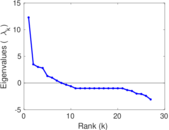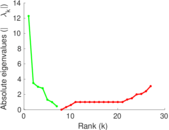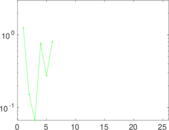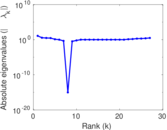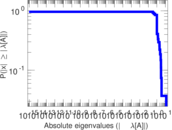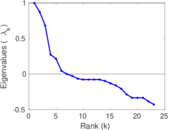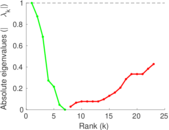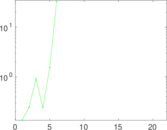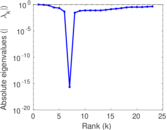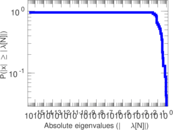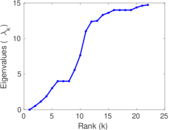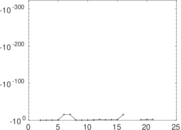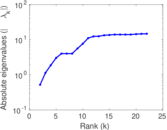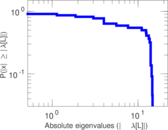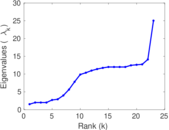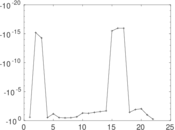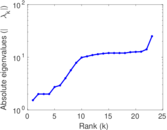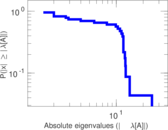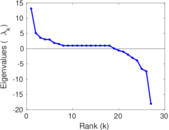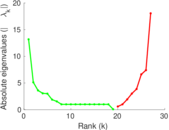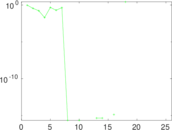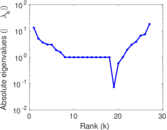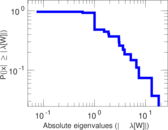# Zebra

This undirected network contains interactions between 28 Grévy's zebras (Equus grevyi) in Kenya. A node represents a zebra and an edge between two zebras shows that there was an interaction between them during the study.

 Code `MZ` Internal name `moreno_zebra` Name Zebra Data source http://moreno.ss.uci.edu/data.html#zebra AvailabilityDataset is available for download Consistency checkDataset passed all tests Category Animal network Dataset timestamp 2007 Node meaning Zebra Edge meaning Interaction Network format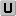Unipartite, undirected Edge typeUnweighted, no multiple edges LoopsDoes not contain loops

## Statistics

 Size n = 27 Volume m = 111 Loop count l = 0 Wedge count s = 1,108 Claw count z = 3,818 Cross count x = 9,291 Triangle count t = 312 Square count q = 2,325 4-Tour count T4 = 23,254 Maximum degree dmax = 14 Average degree d = 8.222 22 Fill p = 0.316 239 Size of LCC N = 23 Diameter δ = 4 50-Percentile effective diameter δ0.5 = 1.197 06 90-Percentile effective diameter δ0.9 = 2.650 39 Median distance δM = 2 Mean distance δm = 1.789 47 Gini coefficient G = 0.307 641 Balanced inequality ratio P = 0.355 856 Relative edge distribution entropy Her = 0.943 726 Power law exponent γ = 1.841 23 Tail power law exponent γt = 1.691 00 Tail power law exponent with p γ3 = 1.691 00 p-value p = 0.000 00 Degree assortativity ρ = +0.717 703 Degree assortativity p-value pρ = 1.969 94 × 10−36 Clustering coefficient c = 0.844 765 Spectral norm α = 12.285 3 Algebraic connectivity a = 0.519 981 Spectral separation |λ1[A] / λ2[A]| = 3.514 66 Non-bipartivity bA = 0.748 511 Normalized non-bipartivity bN = 0.572 364 Algebraic non-bipartivity χ = 1.527 21 Spectral bipartite frustration bK = 0.041 816 5 Controllability C = 1 Relative controllability Cr = 0.037 037 0

## Plots

### Fruchterman–Reingold graph drawing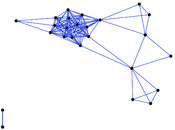### Degree distribution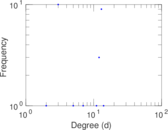### Cumulative degree distribution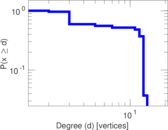### Lorenz curve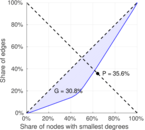### Spectral distribution of the adjacency matrix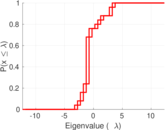### Spectral distribution of the normalized adjacency matrix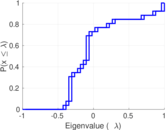### Spectral distribution of the Laplacian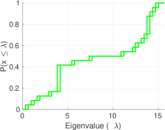### Spectral graph drawing based on the adjacency matrix### Spectral graph drawing based on the Laplacian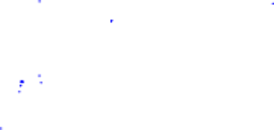### Spectral graph drawing based on the normalized adjacency matrix### Degree assortativity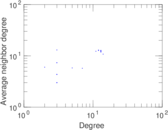### Zipf plot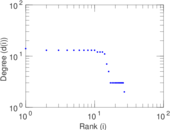### Hop distribution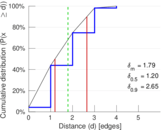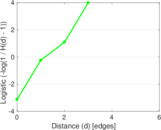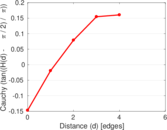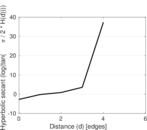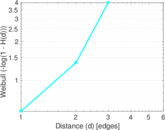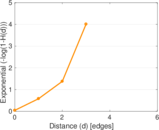### Double Laplacian graph drawing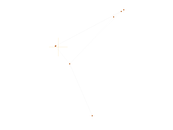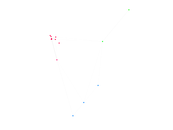### Delaunay graph drawing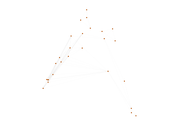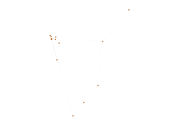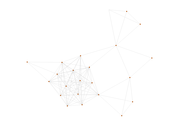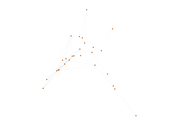### Clustering coefficient distribution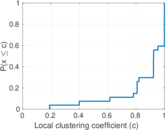### Average neighbor degree distribution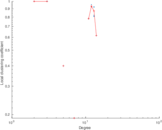### SynGraphy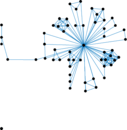### Matrix decompositions plots